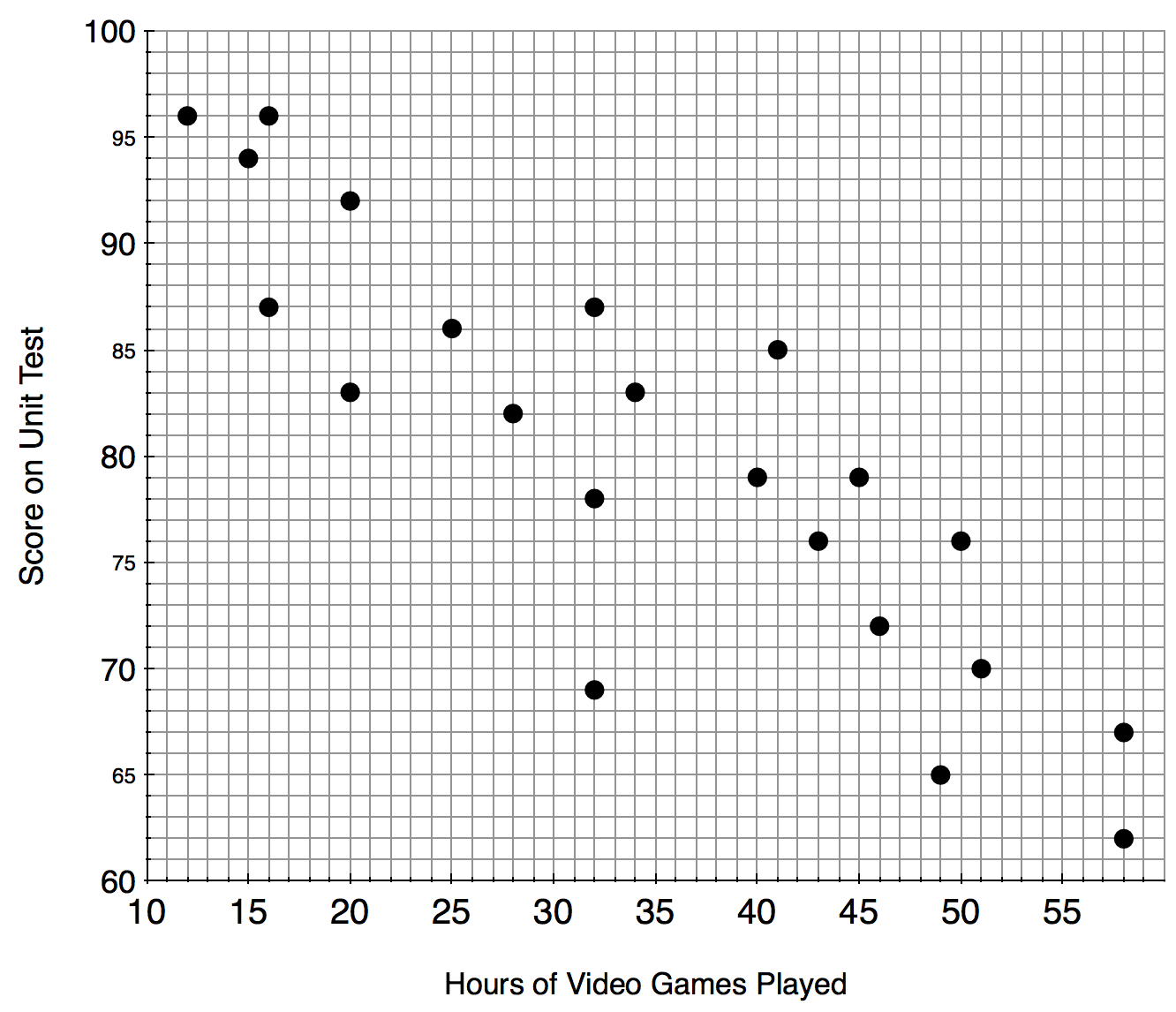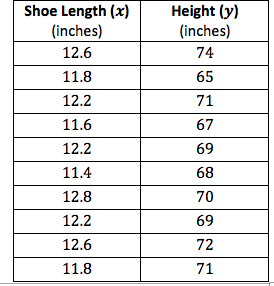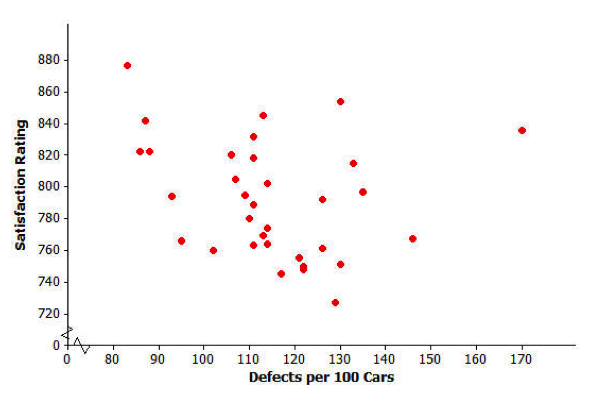# Descriptive Statistics

## Objective

Calculate, with technology, the correlation coefficient for a data set. Explain why correlation does not determine causation.

## Common Core Standards

### Core Standards

?

• HSS-ID.C.8 — Compute (using technology) and interpret the correlation coefficient of a linear fit.

• HSS-ID.C.9 — Distinguish between correlation and causation.

?

• 8.SP.A.2

• 8.SP.A.3

## Criteria for Success

?

1. Explain that the correlation coefficient quantifies the strength and direction of association between two variables.
2. Describe the general categories of correlation—the strength (strong, moderate, and weak) and kind of association (positive/negative).
3. Use the correlation coefficient to explain the strength of correlation. Identify |0.1|-|0.3| as a weak correlation, |0.4|-|0.6| as a moderate correlation, and |0.7|-|0.9| as a strong correlation. 0 is no correlation, and |1.0| is perfect correlation.
4. Explain that just because two variables are correlated does NOT mean that one variable causes the other to happen.
5. Explain the limits of the correlation coefficient—i.e., that the correlation coefficient only describes how closely the points are related linearly, not in any other function type.

## Tips for Teachers

?

• This lesson comes before assigning a line of best fit because the correlation between the variables is measured by the individual point relationships, not the function that is assigned to model the relationship.
• You may want to consider having students develop a bank of Excel formulas useful to describing bivariate data (as with univariate data).

## Anchor Problems

?

### Problem 1

Below is a scatterplot comparing the number of hours of video games played over the course of a month and the score on the unit test.### Problem 2

Use the Desmos activity shown below to answer the question:
Desmos, Andrew Knauft, “Correlation and Regression.”

How does the correlation coefficient help us to quantify the strength of correlation between two variables?

#### References

Correlation and Regression by is made available by Desmos. Copyright © 2017 Desmos, Inc. Accessed June 21, 2017, 11:16 a.m..

### Problem 3

Identify the calculation coefficient, and describe what this means in terms of how the variables are related.## Problem Set

?

The following resources include problems and activities aligned to the objective of the lesson that can be used to create your own problem set.

?

The scatterplot below displays data on the number of defects per  cars and a measure of customer satisfaction (on a scale from  to , with higher scores indicating greater satisfaction) for the  brands of cars sold in the United States in 2009.Data Source: USA Today, June 16, 2010, and July 17, 2010

1. Which of the following is the value of the correlation coefficient for this data set?

r = -0.95

r = -0.24

r = 0.83

r = 1.00

1.  Explain why you selected this value.